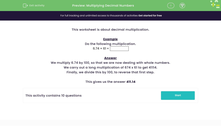# Multiply Decimal Numbers

In this worksheet, students will practise a range of multiplications with decimals of one place, decimals of two places and whole numbers.Key stage:  KS 3

Curriculum topic:   Number

Curriculum subtopic:   Use Four Operations for All Numbers

Popular topics:   Decimals worksheets, Numbers worksheets

Difficulty level:#### Worksheet Overview

This activity is about decimal multiplication.

Example

Do the following multiplication.

 6.74 × 61 =

We multiply 6.74 by 100, so that we are now dealing with whole numbers.

We carry out a long multiplication of 674 x 61 to get 41,114.

Finally, we divide this by 100, to reverse that first step.

This gives us the answer 411.14

Alternatively, we can remove the decimal point, do the calculation, and then add the decimal point back in. Make sure that you have the same number of decimal places in your answer as in the original calculation. In this case, that is two decimal places, giving us 411.14

### What is EdPlace?

We're your National Curriculum aligned online education content provider helping each child succeed in English, maths and science from year 1 to GCSE. With an EdPlace account you’ll be able to track and measure progress, helping each child achieve their best. We build confidence and attainment by personalising each child’s learning at a level that suits them.

Get started